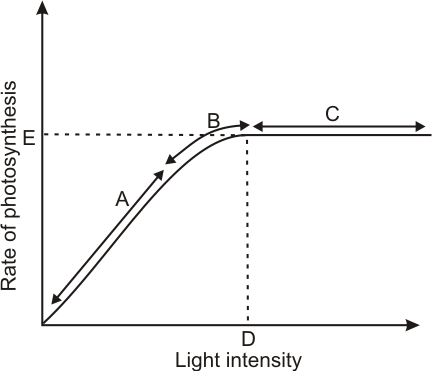Q

# Figure 13.10 shows the effect of light on the rate of photosynthesis. Based on the graph, answer the following questions: (b) What could be the limiting factor s in region A?

8.     Figure 13.10 shows the effect of light on the rate of photosynthesis. Based on the graph, answer the following questions:

(b)Figure 13.10  Graph of light intensity on the rate of photosynthesis

(b) What could be the limiting factor/s in region ?